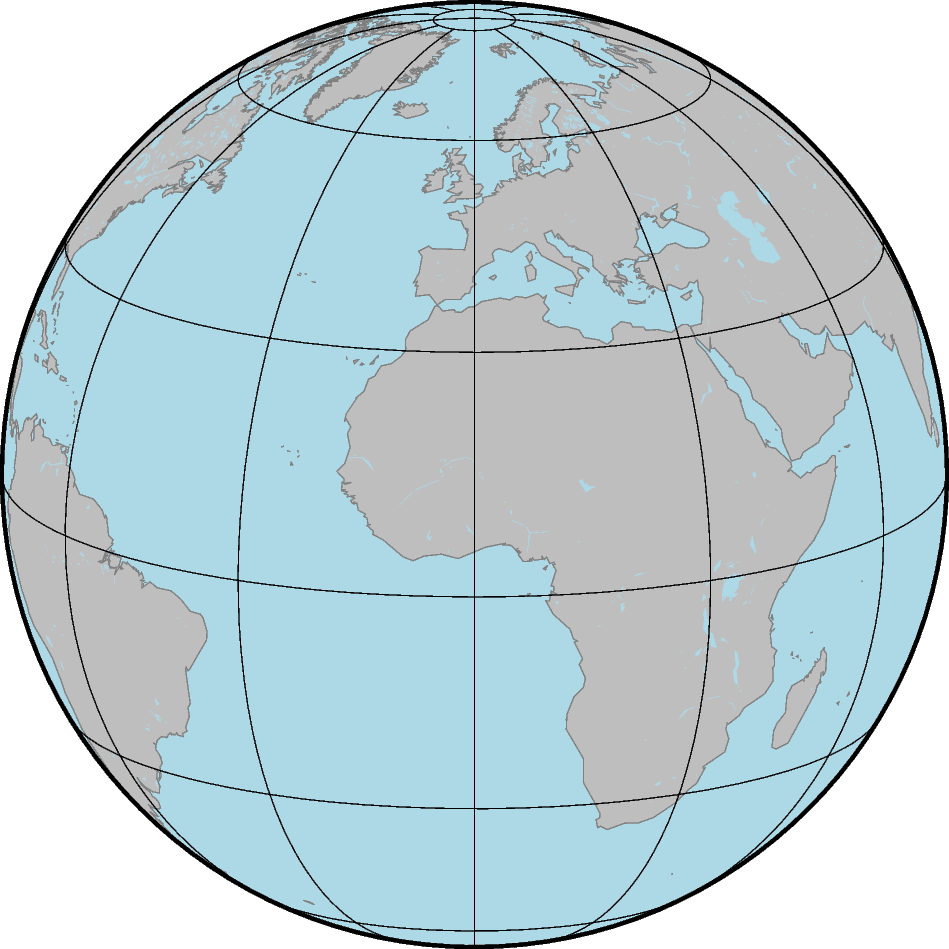# Interactive data visualization using `Panel`

Note

Please run the following code examples in a notebook environment otherwise the interactive parts of this tutorial will not work. You can use the button “Download Jupyter notebook” at the bottom of this page to download this script as a Jupyter notebook.

The library Panel can be used to create interactive dashboards by connecting user-defined widgets to plots. `Panel` can be used as an extension to Jupyter notebook/lab.

This tutorial is split into three parts:

• Make a static map

• Make an interactive map

• Add a grid for Earth relief

```# Import the required packages
import numpy as np
import panel as pn
import pygmt

pn.extension()
```

## Make a static map

The Orthographic projection can be used to show the Earth as a globe. Land and water masses are filled with colors via the `land` and `water` parameters of `pygmt.Figure.coast`, respectively. Coastlines are added using the `shorelines` parameter.

```# Create a new instance or object of the pygmt.Figure() class
fig = pygmt.Figure()
fig.coast(
# Orthographic projection (G) with projection center at 0° East and
# 15° North and a width of 12 centimeters
projection="G0/15/12c",
region="g",  # global
frame="g30",  # Add frame and gridlines in steps of 30 degrees on top
land="gray",  # Color land masses in "gray"
water="lightblue",  # Color water masses in "lightblue"
# Add coastlines with a 0.25 points thick pen in "gray50"
shorelines="1/0.25p,gray50",
)
fig.show()
```## Make an interactive map

To generate a rotation of the Earth around the vertical axis, the central longitude of the Orthographic projection is varied iteratively in steps of 10 degrees. The library `Panel` is used to create an interactive dashboard with a slider (works only in a notebook environment, e.g. Jupyter notebook).

```# Create a slider
slider_lon = pn.widgets.DiscreteSlider(
name="Central longitude",  # Give name for quantity shown at the slider
options=list(np.arange(0, 361, 10)),  # Range corresponding to longitude
value=0,  # Set start value
)

# Define a function for plotting the single slices
@pn.depends(central_lon=slider_lon)
def view(central_lon):
# Create a new instance or object of the pygmt.Figure() class
fig = pygmt.Figure()
fig.coast(
# Vary the central longitude used for the Orthographic projection
projection=f"G{central_lon}/15/12c",
region="g",
frame="g30",
land="gray",
water="lightblue",
shorelines="1/0.25p,gray50",
)
return fig

# Make an interactive dashboard
pn.Column(slider_lon, view)
```
```Column
 DiscreteSlider(formatter='%d', name='Central longitude', options=[0, 10, 20, 30, ...], value=0)
 ParamFunction(function, _pane=PNG)
```

## Add a grid for Earth relief

Instead of using colors as fill for the land and water masses a grid can be displayed. Here, the Earth relief is shown by color-coding the elevation.

```# Download a grid for Earth relief with a resolution of 10 arc-minutes

# Create a slider
slider_lon = pn.widgets.DiscreteSlider(
name="Central longitude",
options=list(np.arange(0, 361, 10)),
value=0,
)

# Define a function for plotting the single slices
@pn.depends(central_lon=slider_lon)
def view(central_lon):
# Create a new instance or object of the pygmt.Figure() class
fig = pygmt.Figure()
# Set up a colormap for the elevation in meters
pygmt.makecpt(
cmap="oleron",
# minimum, maximum, step
series=[int(grd_relief.data.min()) - 1, int(grd_relief.data.max()) + 1, 100],
)
# Plot the grid for the elevation
fig.grdimage(
projection=f"G{central_lon}/15/12c",
region="g",
cmap=True,  # Use colormap defined above
frame="g30",
)
# Add a horizontal colorbar for the elevation
# with annotations (a) in steps of 2000 and ticks (f) in steps of 1000
# and labels (+l) at the x-axis "Elevation" and y-axis "m" (meters)
fig.colorbar(frame=["a2000f1000", "x+lElevation", "y+lm"])
return fig

# Make an interactive dashboard
pn.Column(slider_lon, view)
```
```Column
 DiscreteSlider(formatter='%d', name='Central longitude', options=[0, 10, 20, 30, ...], value=0)
 ParamFunction(function, _pane=PNG)
```

Total running time of the script: ( 0 minutes 3.141 seconds)

Gallery generated by Sphinx-Gallery